# NCERT Solution Exercise – 6.3 Class-10 Maths

Q.1 State which pairs of tringles in fig. 6.34 are similar. write the similarity criterion used by you for answering the question and also write the pairs of similar triangles in the symbolic.

(i)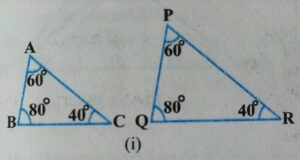Solution:

In Δ ABC and Δ PQR

∠A =∠P =60°

∠B =∠Q =80°

∠C=∠R =40°

∴ Δ ABC ∼ Δ PQR [∴Δ AAA similarity]

(ii)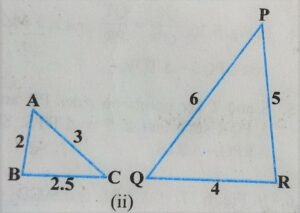Solution:

In Δ ABC and Δ QRP

AB/QR = 2/4 = 1/2

BC/RP = 2.5/5×10 =1/2

AC/PQ = 3/6 = 1/2

∴ AB/QR=BC/RP=AC/PQ=1/2

(iii)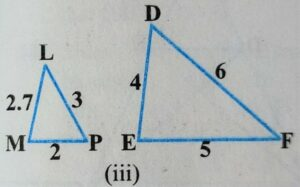Solution:

In Δ LMN and Δ DEF

MN/DE =2/4=1/2

LN/DF =3/6=1/2

LN/EF =2.7/5

MN/DE=LN/DF≠LM/EF

1/2=1/2≠2.7/5

now LMN & not similar to DEF

(iv)Solution:

In Δ MNL and PQR

∠M = ∠Q

ML/QR = 5/20 = 1/2

MN/PQ = 2.5/60 = 5/12

ML/QR ≠ MN/PQ

now ΔMNL is not similar Δ PQR

(v)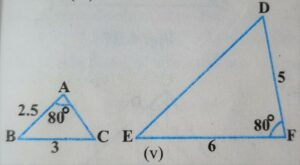Solution:

Δ ABC and Δ DEF

∠A = ∠F = 80°

AB/DF = 2.5/5×10 = 1/2

BC/EF = 3/6 = 1/2

now

AB/DF = BC/EF =1/2

Δ ABC∼ Δ DEF [∴SAS similarity]

(vi)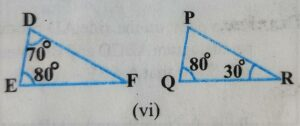Solution:

∠Q=∠E=80°

∠R=∠F=30°

∠P=∠D

ΔPQR∼ΔDEF[∴AAA similarity]

Q.2 In fig. 6.35, ΔODC∼ΔOBA,∠BOC=125°and∠CDO=70°,find ∠DOC,∠DCOand∠OAB.

Given:

In Δ ODC  and Δ OBA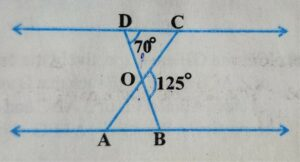Δ ODC ∼ Δ OBA

∠BOC=125° and
∠CDO=70°

To find:

∠DOC=?

∠DCO=?

∠OAB=?

Solution:

In ΔODC and ΔOBA

ΔODC ∼ ΔOBA [given]

∴ corresponding angles are also equal.

∠ODA=∠OBA=70°

∠DOC+∠COB=180°  [linear pair angles]

⇒ ∠DOC+125°=180°

⇒ ∠DOC=180°-125°

⇒ ∠DOC = 55°

In Δ DOC

∠DOC+∠CDO+∠DCO=180°[∴angle sum property Δ]

⇒ 55°+70°+∠DCO=180°

⇒ 125°+∠DCO=180°

⇒ ∠DCO=180°-125°

⇒ ∠DCO=55°————–(1)

∴ ∠OAB = ∠DCO [ ∴corresponding angles are equal in similar Δ ]

∴ ∠OAB = 55° [ from equation(1) ]

Q.3 Diagonals AC and BD of a trapezium   ABCD with AB//DC intersect each other at the point O. Using a similarity criterion for two triangles, show that  OA/OC=OB/OD

Given:

In trapezium ABCD

AB II DC

To Show: using the similarity criterion two triangles

OA/OC = OB/OD

Proof:

In trapezium ABCD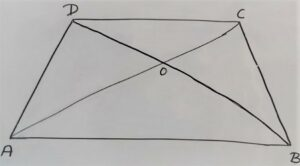AB II DC

In Δ DOC and Δ BOA

∠CDO = ∠ABO (∴ Alternate interior angle →A.I.A)

∠COD = ∠AOB (∴ vertically opposite angles )

∠OAB = ∠OCD (∴ Alternate interior angle →A.I.A)

∴ ΔDOC ∼ Δ BOA (∴ AAA similarity  criterion )

corresponding sides ratio is also equal.

AO/OC = OB/OD   hence prove

Q,4 In fig 6.36 QR/QS = QT/PR and ∠1 = ∠2 show that ΔPQS∼ΔTQR

Given: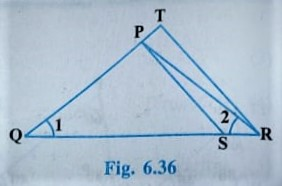ΔQRT

QR/QS = QT/PR

∠1=∠2

To Show:

ΔPQR ∼ ΔTQR

Proof:

In ΔPQR

∠1 = ∠2 [∴ given ]

∴PQ = PR [ equal angles of opposite sides also equal ] —–(1)

QR/QS = QT/PR [∴given]

⇒ QR/QS = QT/PQ

⇒ QS/QR = PQ/QT —–(2)

In ΔPQS and ΔTQR

∠1 = ∠1 [∴common angle ]

QS/QR = PQ/QT  [∴ from equation 2]

∴ Δ PQS ∼ ΔTQR [ by SAS similarity ]

Hence proved.

Q.5 S and T are points on sides PR and QR of ΔPQR such that ∠P =∠RTS show that ΔRPQ∼ΔRTS

Given: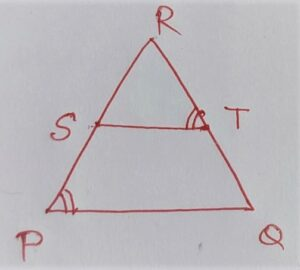In Δ PQR

S and T are on sides PR and QR

∠P = ∠RTS

To Show:

ΔRPQ ∼ΔRTS

Proof:

In Δ RPQ and ΔRTS

∠RPQ = ∠RTS [∴given]

∠PRQ = ∠TRS [∴ common angle]

∴ ΔRPQ  ∼ ΔRTS [by AA similarity]

Q.6 In fig. 6.37, if ΔABE ≅ ΔACD, show that ΔADE∼ΔABC

Given:  Δ ABE ≅ ΔACD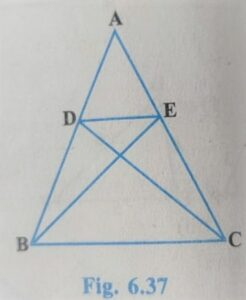Proof:

In ΔABE and ΔACD ÷

ΔABE ≅ ΔACD

AB = AC [ by cpct ]

AE = AD [ by cpct ]

⇒ AB/Ac =1

⇒ AB/AC = AE/AD = 1

In Δ ADE and Δ ABC

∠A = ∠A [ ∴ common angle ]

AB/AE = AC/AD [ from equation (1) ]

∴ Δ ADE ∼ Δ ABC [ SAS similarity]

Hence Proved.

Q.7 In Fig. 6.38, altitudes AD and CE of ΔABC intersect each other at point p show that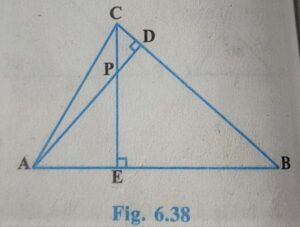(I) ΔAEP ∼Δ CDP

Solution:

In ΔAEP and ΔCDP

∠APE = ∠CPD [∴ vertically opposite angle ]

∠AEP = ∠CDP = 90° [∴ given ]

∴ ΔAEP ∼ ΔCDP [ AA similarity criterion ]

(II) ΔABD ∼ΔCBE

Solution:

Δ ABC and Δ CBE

∠B = ∠B [∴ common angle ]

∠ADB = ∠CED = 90° [∴ given]

Δ ABD ∼ ΔCBE [∴AA similarity criterion ]

Solution:

∠PAE = ∠DAB [∴ common angle ]

∠AEP = ∠ADB = 90° [∴AA similarity ]

(IV)  In Δ PDC and ΔBEC

Solution:

∠PCD = ∠ECB [ ∴ common]

∠COP = ∠CEB = 90° [∴given]

ΔPDC∼ΔBEC [ AA similarity ]

Q.8 E is a point on the side of AD produced by a parallelogram ABCD and BE intersects CD at F. This shows that ΔABE∼ΔCFB.

Given: In parallelogram ABCD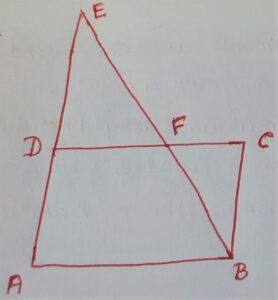∠A = ∠C

To prove: ΔABE∼ΔCFB

Proof:  In  ΔABE and ΔCFB

∠A = ∠C [∴ given]

∠ABE=∠CFB [ alternate  interior angle ]

ΔABE ∼ ΔCFB [ AA similarity creterion]

Q.9 In Fig. 6.39, ABC and AMP are two-night triangles, night angled at B and M respectively. prove that :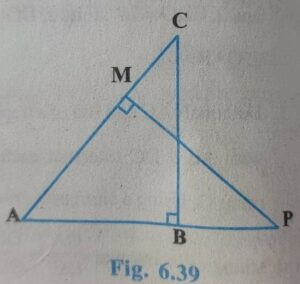(i)ΔABC∼ΔAMP

Solution:

In ΔABC and ΔAMP

∠ABC=∠AMP=90° [given]

∠A=∠A [∴ common angle ]

ΔABC∼ΔAMP [∴ AA similarity criterion ]

(ii) CA/PA = BC/MP

Solution:

CA/PA = BC/MP [ criterion of similarity ]

Q.10 CD and GH are respectively the bisectors of ∠ACB and ∠EGF such that D and H lie on sides AB and FE of ΔABC and ΔEFG respectively. If ΔABC∼ΔFEG, show that :

(i) CD/GH = AC/FG

(ii) ΔDCB ∼ ΔHGE

(iii) ΔDCA ∼ ΔHGF

Given: In ΔABC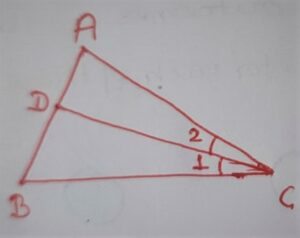∠1=∠2 [ CD bisector of ∠c ]

In ΔEFG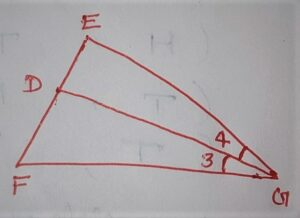∠3=∠4 [ GH is bisector ∠g ]

ΔABC ∼ ΔFEG

To show:

(i) CD/GH = AC/FG

(ii) ΔDCB ∼ ΔHGE

(iii) ΔDCA ∼ ΔHGF

Solution:

ΔABC ∼ ΔFEG [∴ given ]

∠C = ∠G [∴ by similarity criterion ]

⇒ 1/2 ∠C =1/2 ∠G

⇒ ∠1=∠3 or ∠2=∠4

∠1=∠4

∠2=∠3

now In ΔACD and ΔFGH

∠A=∠F [∴ given ΔABC∼ΔFEG ]

∠2=∠3

ΔACD ∼ΔFGH [ AA similarity ]

CD/GH = AC/FG [ by similarity criterion ]

(ii) In ΔDCB ∼ ΔHGE

Solution:

∠1=∠4 [proved]

∠B= ∠E [∴ given ΔABC∼ΔFEG]

ΔDCB∼ΔHGE [AA similarity ]

(iii) In ΔDCA and ΔHGE

∠A=∠F [∴ given ] [∴ΔABC∼ΔFEG]

∠2=∠3 [∴ proved]

ΔDCA∼ΔHGE [∴AA similarity]

Q.11 In fig 6.40, E is a point on side EB produced of an isosceles triangle ABC with AB=AC. if AD⊥BC and EF⊥AC, prove that ΔABD∼ΔECF.

Given: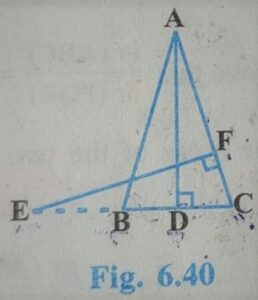In ΔABC

AB=AC

∠B=∠C

EF⊥AC

To prove:

ΔABD∼ΔECF

Proof:

In ΔABD and ΔECF

∠ABD=∠EFC=90° [∴given]

∠B=∠C [given equal sides of the opposite angles are also equal in an isosceles triangle]

ΔABD∼ΔECF [ AA similarity ]

Hence Proved.

Q.12 sides AB and BC and median AD of a triangle ABC are respectively proportional to sides PQ and QR and the median PM of ΔPQR (see 6.41). Show that  ΔABC∼ΔPQR.

Given: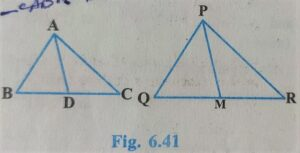In ΔABC and ΔPQR

To show:

ΔABC∼ΔPQR

Proof:

In ΔABD and ΔPOM

D and M are the midpoints of BC and QR respectively

∴ BD=DC     ;  QM=MR

2BD=BC     ; 2QM=MR=QR

ΔABD∼ΔPOM [∴SSS similarity ]

∠B = ∠Q [ by similarity criterion ] ——–(1)

In ΔABC and ΔPQR

AB/PQ=BC/QR [∴given]

∠B=∠Q [proved in equation (1)]

ΔABC∼ΔPQR [ SAS similarity]

Q.13 D is a point on the side BC of a triangle ABC such that ∠ADC =∠BAC show that CA²= CB. CD

Given:

In ΔABC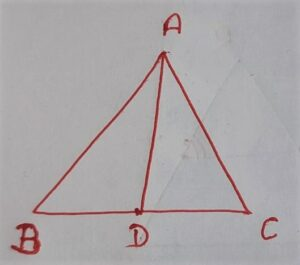D is a point on side BC

To show:

CA² = CB.CD

Proof:

In ΔABC and ΔDAC

∠C=∠C [∴ common angle ]

ΔABC ∼ ΔDAC [ AA similarity]

CA/CD = CB/CA [ by similarity criterion]

⇒ CA.CA = CB.CD

⇒ CA² = CB.CD

Q.14 sides AB and AC and median Ad of a triangle ABC are respectively proportional to sides PQ and PR and median PM of another triangle PQR show that ΔABC∼ΔPQR.

Given:

In ΔABC and ΔPQR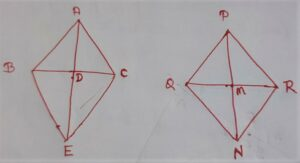To show:

ΔABC∼ΔPQR

Construction:

In ΔABC:

Produce AD to E such that AD = DE join BE and CE

In ΔPQR:

Produce PM to N such that PM = MN join N and RN

Proof:

In Quadrilateral ABEC Diagonals bisect each other at point D

∴ ABCD is a parallelogram.

∴ BE=AC ——(2)

Similarly In quadrilateral PQNR: diagonals are bisect each other at point M

∴ PQNR is parallelogram

QN = PR ———(3)

equation (2) ÷equation(3)

BE/QN = AC/PR ———(4)

by equation (1)  (4) & (5)

In ΔABE and ΔPQN

AB/PQ=BE/QN=AE/PN [ by equation (6) proved ]

ΔABE∼ΔPQN [by SSS similarity]

∴ ∠BAE = ∠QPN————(7)

similarily ΔCAE ∼ ΔRPN———–(8)

equation (7) + equation (8)

∠BAE+∠CAF=∠QPN+∠RPN

⇒ ∠A=∠P——–(9)

Now In ΔABC and ΔPQR

AB/PQ=AC/PR [∴given]

∠A=∠P [∴by equation(9) proved ]

ΔABC∼ΔPQR [ SAS similarity ]

Q.15 A vertical pole of length 6m casts a shadow 4m long on the ground and at the same time a tower casts a shadow 28m long. find the height of the tower.

Solution:

In ΔABC and ΔPQR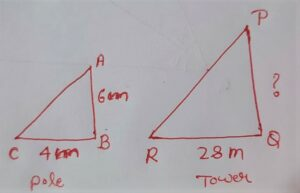∠B=∠Q=90°

∠C=∠R [∴At the same time angle of the shadow is also the same ]

ΔABC∼ΔPQR

∴AB/PQ=BC/QR=AC/PR [by similarity criterion]

⇒ AB/PQ=BC/QR

ΔABC∼ΔPQR

∴ AB/PQ=BC/QR=AC/PR [by similarity criterion]

⇒ AB/PQ=BC/QR

⇒ 6/x=4/28

⇒ 6×28=4x

⇒ 6×28/4=x

⇒ 6×7=x

⇒ 42m=x

Hence the height of the tower is 42m.

Q.16 If AD and PM are medians of triangles ABC and PQR, respectively where ΔABC∼ΔPQR, prove that AB/PQ=AD/PM

Given:

In ΔABC and  ΔPQR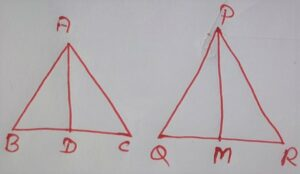PM=median

ΔABC∼ΔPQR

To Prove:

Proof:

ΔABC∼ΔPQR [∴given]

∠B =∠Q [∴by similarity criterion]

In ΔABD and ΔPQM

AB/PQ=BC/QR [ given ΔABC∼ΔPQR]

⇒ AB/PQ=2BD/2QM [∴ BD=CD & QM=RM]

⇒ AB/PQ=BD/QM

⇒ ∠B=∠Q [ given ΔABC∼ΔPQR]

ΔABD∼ΔPQM [∴ SAS similarity ]

∴ AB/PQ=AD/PM [ by similarity criterion]

Hence proved.